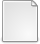# Higher Order Frechet Derivatives of Matrix Functions and the Level-2 Condition Number

Higham, Nicholas J. and Relton, Samuel D. (2014) Higher Order Frechet Derivatives of Matrix Functions and the Level-2 Condition Number. SIAM Journal on Matrix Analysis and Applications, 35 (3). pp. 1019-1037. ISSN 1095-7162PDF 130945259.pdf Download (253kB)Other mfiles.zip Download (2kB)
Official URL: http://epubs.siam.org/doi/abs/10.1137/130945259

## Abstract

The Fr\'echet derivative $L_f$ of a matrix function $f \colon \mathbb{C}^{n\times n} \mapsto \mathbb{C}^{n\times n}$ controls the sensitivity of the function to small perturbations in the matrix. While much is known about the properties of $L_f$ and how to compute it, little attention has been given to higher order Fr\'echet derivatives. We derive sufficient conditions for the $k$th Fr\'echet derivative to exist and be continuous in its arguments and we develop algorithms for computing the $k$th derivative and its Kronecker form. We analyze the level-2 absolute condition number of a matrix function (the condition number of the condition number'') and bound it in terms of the second Fr\'echet derivative. For normal matrices and the exponential we show that in the 2-norm the level-1 and level-2 absolute condition numbers are equal and that the relative condition numbers are within a small constant factor of each other. We also obtain an exact relationship between the level-1 and level-2 absolute condition numbers for the matrix inverse and arbitrary nonsingular matrices, as well as a weaker connection for Hermitian matrices for a class of functions that includes the logarithm and square root. Finally, the relation between the level-1 and level-2 condition numbers is investigated more generally through numerical experiments.

Item Type: Article matrix function, Frechet derivative, Gateaux derivative, higher order derivative, matrix exponential, matrix logarithm, matrix square root, matrix inverse, matrix calculus, partial derivative, Kronecker form, level-2 condition number, expm, logm, sqrtm, MATLAB MSC 2010, the AMS's Mathematics Subject Classification > 15 Linear and multilinear algebra; matrix theoryMSC 2010, the AMS's Mathematics Subject Classification > 65 Numerical analysis Nick Higham 16 Apr 2015 20 Oct 2017 14:13 http://eprints.maths.manchester.ac.uk/id/eprint/2160

### Available Versions of this ItemView Item